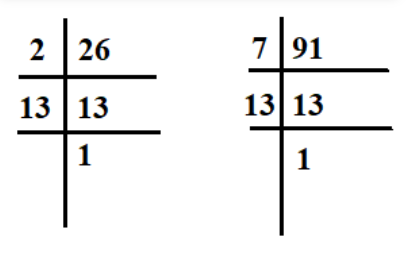Home/Class 10/Maths/

Find the LCM and HCF of $$26$$and $$91$$ and verify that LCM $$\times$$ HCF $$=$$Product of two numbersSpeed
00:00
03:00QuestionMathsClass 10

Find the LCM and HCF of $$26$$and $$91$$ and verify that LCM $$\times$$ HCF $$=$$Product of two numbers

4.64.6Solution

Solution:
Doing prime factorization of $$26$$ and $$91$$.$$26=2\times 13$$ and $$91=7\times 13$$
$$\therefore$$ LCM of $$26$$ and $$91$$ $$=2\times 7\times 13=182$$
and HCF of $$26$$ and $$91 =13$$
Now,
LCM $$\times$$HCF$$=182\times 13=2366$$
Product of two numbers$$=26\times 91=2366$$
Since,$$182\times 13=26\times 91$$
so,  LCM $$\times$$ HCF $$=$$Product of two numbers
Hence proved.